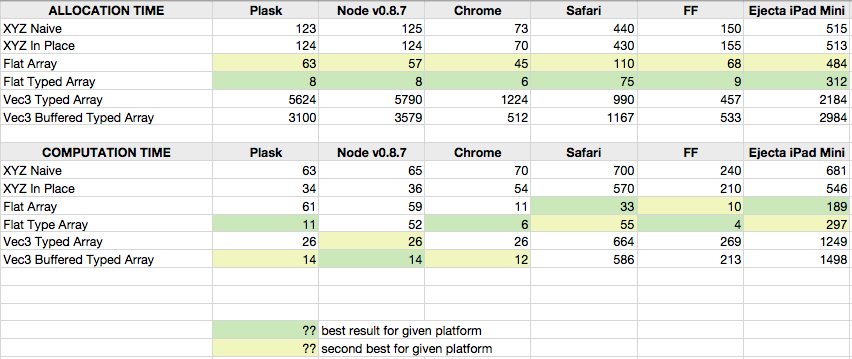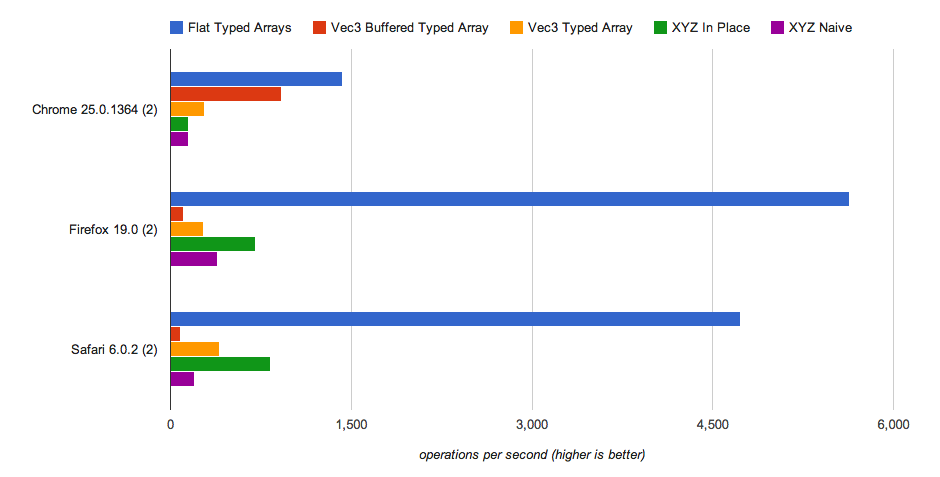2013-03-18

# Fast Dynamic Geometry in WebGLRecently I do a lot of WebGL programming that involves dynamic generative geometry. One of the problems with JavaScript is it's performance. Generally it's ok as long you don't create too many new object every frame and don't try to push to GPU too much at once. And as always it's a compromise between speed and convenience. Imagine this piece of generating bunch of random points:

``````var positions = [];
for(var i=0; i<1000; i++) {
positions.push(new Vec3(Math.random(), Math.random(), Math.random()));
}

//let's do some operations on our data
for(var i=0; i``````

`In order to display it on the screen you need to upload it to GPU. There is one problem though. Or data is laid out in the memory as array or arrays (list of lists to be exact):`

``````[[x, y, z], [x, y, z], [x, y, z], ...]
``````

`While WebGL want's them as flat array:`

``````[x, y, z, x, y, z, x, y, z, …]
``````

`So at some point in our engine we will have ugly piece of code like this:`

``````var webGLbuffer = new Float32Array(positions.length * 3);
for(var i=0; i``````

`It's fine if you do it once but doing this every frame seems wasteful so there must be another way... What if we started with the right data layout from the beginning.`

``````var positionsData = new Float32Array(1000 * 3);;
var numVertices = positionsData.length/3;
for(var i=0; i 0) {
positions[i  ] /= len;
positions[i+1] /= len;
positions[i+2] /= len;
}
}

var webGLbuffer = positionsData; //no extra work needed
``````

`As you can see you have to choose between short code or speed.`

### `Buffered Vertex Array`

`I'm in the middle of rewriting my WebGL engine at the moment (it's not Three, sorry) and I decided to switch to glMatrix. The code from above is looking now like this:`

``````var positions = [];
for(var i=0; i<1000; i++) {
var p = Vec3.fromValues(Math.random(), Math.random(), Math.random());
Vec3.normalize(p, p);
positions.push(p);
}
``````

`Normalize function and other operations are super fast but allocating 1000 small Float32Arrays is not a very good idea, just see benchmarks below. And in the end we still have problem of flattening our array every frame.`

``````var webGLbuffer = new Float32Array(positions.length * 3);
for(var i=0; i``````

`But when reading Typed Arrays spec is noticed that you can create one many 'views' on top of one underlying buffer. Hmm. That means that we can create one data buffer, and then on top of it one big array for WebGL and many smaller arrays for our vectors.`

``````function Vec3Array(n) {
Array.call(this);
this.length = n;

this.bufStorage = new ArrayBuffer(NUM_ELEMENTS * n * ELEMENT_BYTES);
this.buf = new Float32Array(this.bufStorage, 0, NUM_ELEMENTS * n);
for(var i=0; i``````

`So our code looks now like this:`

``````var positions = new Vec3Array(1000);
for(var i=0; i``````

`Using this we can reduce allocation speed of Typed Arrays vectors, have easy way to upload to WebGL and still have convenient way to perform operations on our vertices. Awesome? It's complicated.`

### `Benchmarking`

`To test how this code actually performs I tried couple of approaches.`

1. `XYZ native - arrays of {x:0, y:0, z:0} objects, naive because operations like a.added(b) create new objects every time they are called`

2. `XYZ in place - arrays of {x:0, y:0, z:0} objects with math operations 'in-place' without creating new objects`

3. `Flat Typed Arrays - flat array of [x, y, z, x, y, z, ...] super fast but annoying to work with as every operation takes three lines of code so instead of Vec3.add(out, a, b) we have to do out = a + b; out = a + b; out = a + b; which obfuscates any slightly more complicated operation. E.g. BufferedGeometry in Three.JS`

4. `Vec3 typed array - arrays of Float32 typed arrays, math operations are done in place but instead of x,y,z properties with have to address each components by index , , , glMatrix approach.`

5. `Vec3 Buffered typed array - arrays of Float32 typed arrays with one common underlying buffer in theory should be as fast with computation as 4. but with even faster upload to GPU (no need to flatten the buffer). In practice is very implementation specific.`

`What I'm actually testing?`

``````//WARNING pseudocode, real code depends on vector implementation

var as = array(100000);
var bs = array(100000);
var cs = array(100000);

for(var i=0; i<100000; i++) {
cs[i] = as[i] + bs[i];
cs[i] = normalized(cs[i]);
cs[i] = cs[i] * 5;
}
``````

`Here are the average results of running each code 10x.`

`As you can see nothing can beat simply flat Float32Array but your computations won't be pretty. And well, creating many Float32Arrays is SLOW. But hopefully you do it only once. I work mostly with Plask and Chrome so this works for me but in general it depends too much on implementation (look at Safari, Firefox and Ejecta).I created jsperf test for it if you would like to try it your self. So far it looks like Safari and Firefox implementations of Typed Arrays are so slow that this approach is unusable. :(You can also download the code vec3array.zip.`